29 C
Jaipur
Saturday, May 8, 2021

# What Is BC in a Bash Script? – Linux Hint

### How Duolingo became an edtech leader – TechCrunch

BC, which stands for Basic Calculator, is a command in Bash that is used to provide the functionality of a scientific calculator within a Bash script. This can be useful for scripting with various arithmentic use cases and scenarios. This article shows you how to use BC in a Bash script.

## Examples of using the BC Command in a Bash Script

To learn more about using the BC command in a Bash script in Linux Mint 20, take a look at the examples provided in the following sections:

## Example 1: Calculating the Power of a Number

Bash programming only allows us to perform mathematical operations on integers, i.e., you cannot perform calculations with decimals or floating-point numbers in Bash. To calculate the power of a decimal with an integer exponent, we will write the following Bash script:

In this Bash script, a one-liner echo command calculates the second power of “2.5.” The result is piped to the BC command, which will perform this calculation.

After saving this script, we can execute the following command: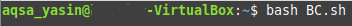The output of our Bash script results in a decimal number, as shown in the image below. This operation would not have been possible without the BC command.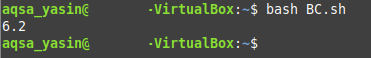## Example 2: Checking Whether a Number is Less than Another Number

The BC command can also be used to compare if a number is greater or less than another. To draw such a comparison, we will write the following Bash script: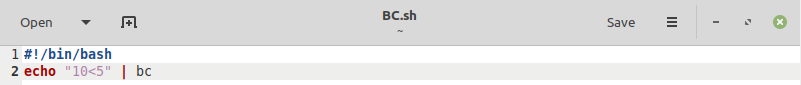In this Bash script, again, a one-liner echo command is used. The command checks whether one number is less than another number. The result is piped to the BC command, which will perform this comparison. The output of this contrast will be “1” if the given statement is true; otherwise, the output will be “0.”

The output of the above Bash script is “0” since 10 is greater than 5, which makes our statement false. This output can be seen in the image below: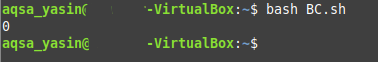## Example 3: Checking Whether a Number Is Equal to Another Number

As in Example 2, the BC command is used again in this example; however, this time, the command will check whether one number is equal to another number. To draw such a comparison, we will write the following Bash script: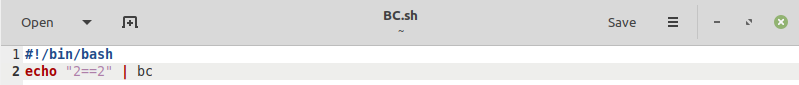In this Bash script, a one-liner echo command checks whether the first number is equal to the other number. The result is piped to the BC command, which will perform this comparison. The output of this script will be “1” if the given statement is true; otherwise, the output will be “0” if the statement is false.

The output of our Bash script is “1” since 2 is equal to 2, which makes our statement true. This output can be seen in the image below: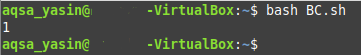## Example 4: Using the BC Command with the && Logical Operator

The BC command can also be paired up with logical operators in Bash, including && and ||, which correspond to logical multiplication and logical addition, respectively. The outcome of the && logical operator is true, or “1,” when all the provided inputs are non-zero. Otherwise, the result will be false, or “0.” To use the && operator with the BC command in Bash, we will write the following Bash script: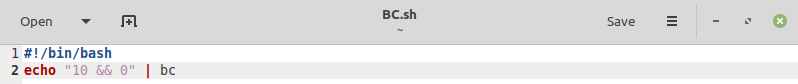In this Bash script, a simple one-liner echo command performs the logical operation && between the numbers “10” and “0.” The result is piped to the BC command that will perform this operation.

The output of our Bash script is “0” since at least one of our provided values is not non-zero, which makes our operation false. This output can be seen in the image below: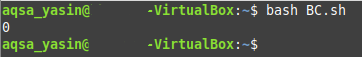## Example 5: Using the BC Command with the || Logical Operator

The result of the || logical operator is true, or “1,” when one of the provided inputs is non-zero. Otherwise, the result will be false, or “0.” To use the || operator with the BC command in Bash, we will write the following Bash script: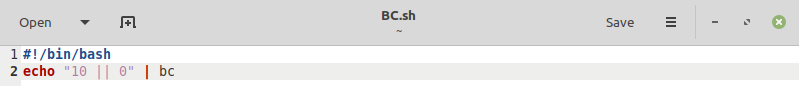In this Bash script, a simple one-liner echo command performs the logical operation || between two numbers, “10” and “0.” The result is piped to the BC command that will perform this operation.

The output of our Bash script is “1” since one of our provided values is non-zero, which makes our operation true. This output can be seen in the image below: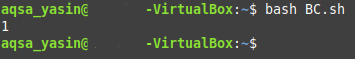## Example 6: Dividing Decimal Numbers with the Result in Decimal

We can also use the BC command to divide decimal numbers and return the result in decimal form, up to a specific decimal place. To obtain this decimal precision, we will write the following Bash script: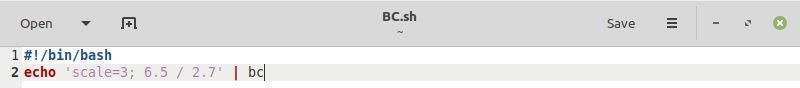In this Bash script, a one-liner echo command divides two numbers, “6.5” and “2.7.” We want the result to be accurate to “3” decimal places, and we have used the “scale” flag for this purpose. The result is piped to the BC command that will perform this calculation.

The output of our Bash script results in a decimal number that is correct up to 3 decimal places, as shown in the image below. This would not have been possible without using the “scale flag” with the BC command. The output can be seen in the image below: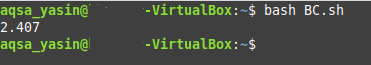## Conclusion

In this article, we provided several examples of using the BC command in Bash script in Linux Mint 20. However, there is still a lot more that you can do with this powerful command that you can explore on your own and add more math and calculations to your bash scripts.